# Arduino Sunflower: An Electronic Sun-Dancer

An electronic sun-dancing sun tracker.

BeginnerFull instructions provided9,467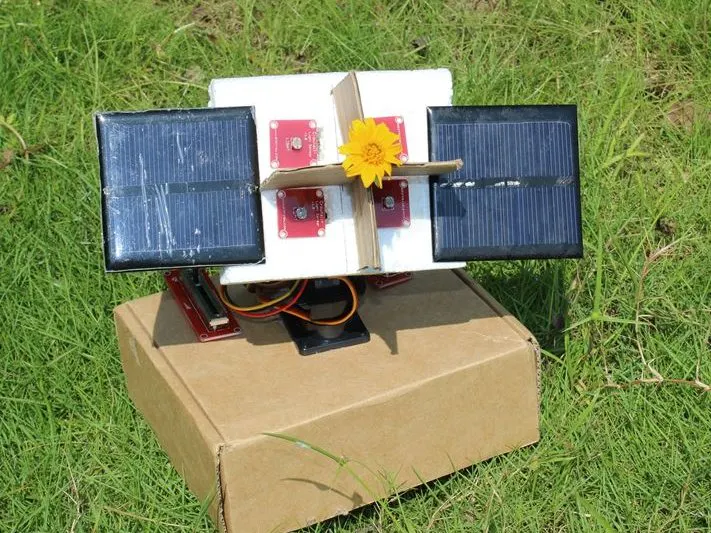## Things used in this project

### Hardware componentsArduino UNO & Genuino UNO
×1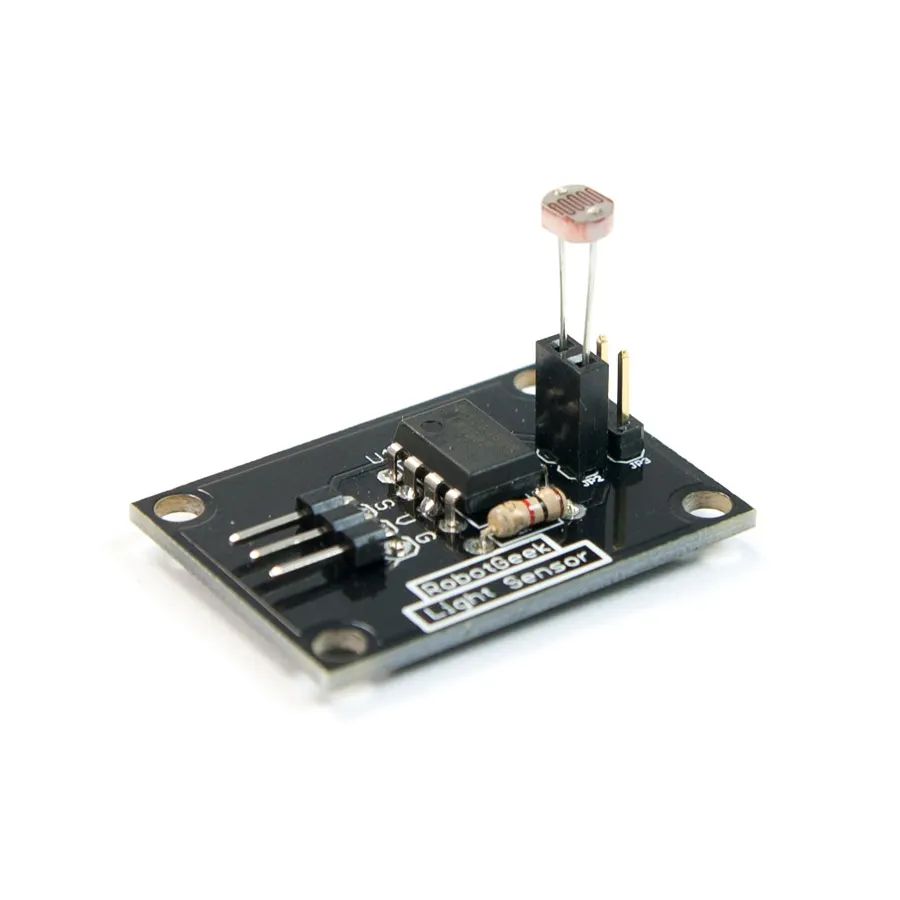RobotGeek Light Sensor
×1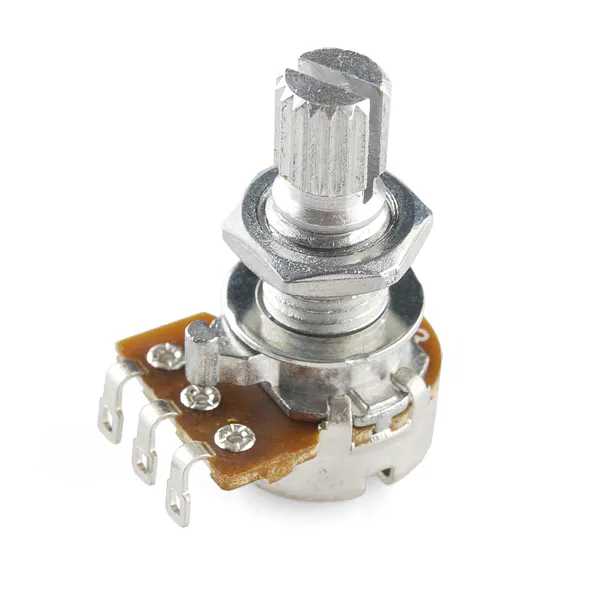Rotary potentiometer (generic)
×1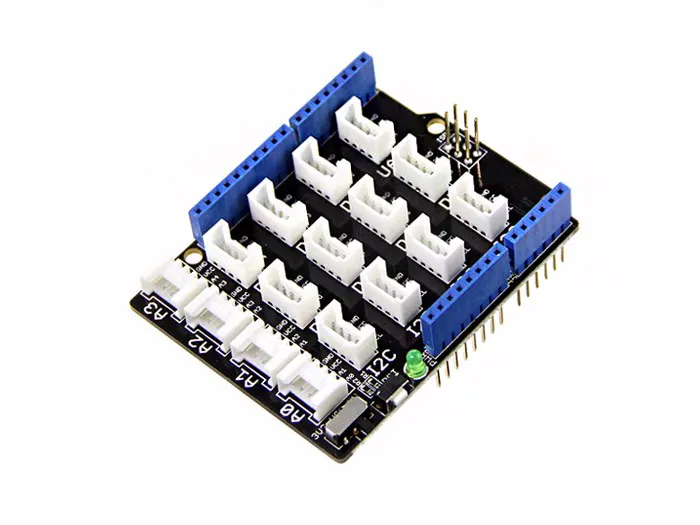Seeed Base Shield V2
×1

### Software apps and online servicesArduino IDE

## Custom parts and enclosures

### CONNECTIONS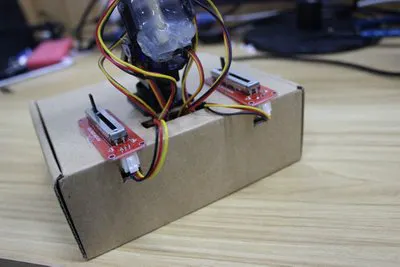## Schematics

### CONNECTIONS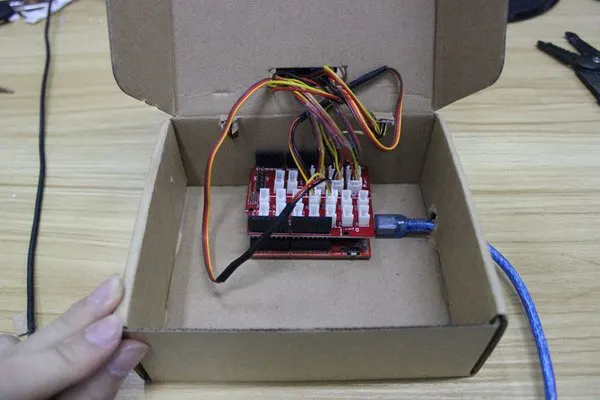## Code

### CODE FOR ARDUINO SUNFLOWER

Arduino
```#include <Servo.h> // include Servo library

Servo horizontal; // horizontal servo
int servoh = 90; // stand horizontal servo

Servo vertical; // vertical servo
int servov = 90; // stand vertical servo

// LDR pin connections
// name = analogpin;
int ldrrd =0;
int ldrld =1;
int ldrlt = 2;
int ldrrt = 3;

void setup()
{
Serial.begin(9600);
// servo connections
// name.attacht(pin);
horizontal.attach(9);
vertical.attach(10);
}

void loop()
{
int lt = analogRead(ldrlt); // top left
int rt = analogRead(ldrrt); // top right
int ld = analogRead(ldrld); // down left
int rd = analogRead(ldrrd); // down rigt

int avt = (lt + rt) / 2; // average value top
int avd = (ld + rd) / 2; // average value down
int avl = (lt + ld) / 2; // average value left
int avr = (rt + rd) / 2; // average value right

int dvert = avt - avd; // check the diffirence of up and down
int dhoriz = avl - avr;// check the diffirence og left and rigt

if (-1*tol > dvert || dvert > tol) // check if the diffirence is in the tolerance else change vertical angle
{
if (avt > avd)
{
servov = ++servov;
if (servov > 180)
{
servov = 180;
}
}
else if (avt < avd)
{
servov= --servov;
if (servov < 0)
{
servov = 0;
}
}
vertical.write(servov);
}

if (-1*tol > dhoriz || dhoriz > tol) // check if the diffirence is in the tolerance else change horizontal angle
{
if (avl > avr)
{
servoh = --servoh;
if (servoh < 0)
{
servoh = 0;
}
}
else if (avl < avr)
{
servoh = ++servoh;
if (servoh > 180)
{
servoh = 180;
}
}
else if (avl == avr)
{
// nothing
}
horizontal.write(servoh);
}
delay(dtime);
}
```

## Credits

### SURYATEJA

18 projects • 33 followers